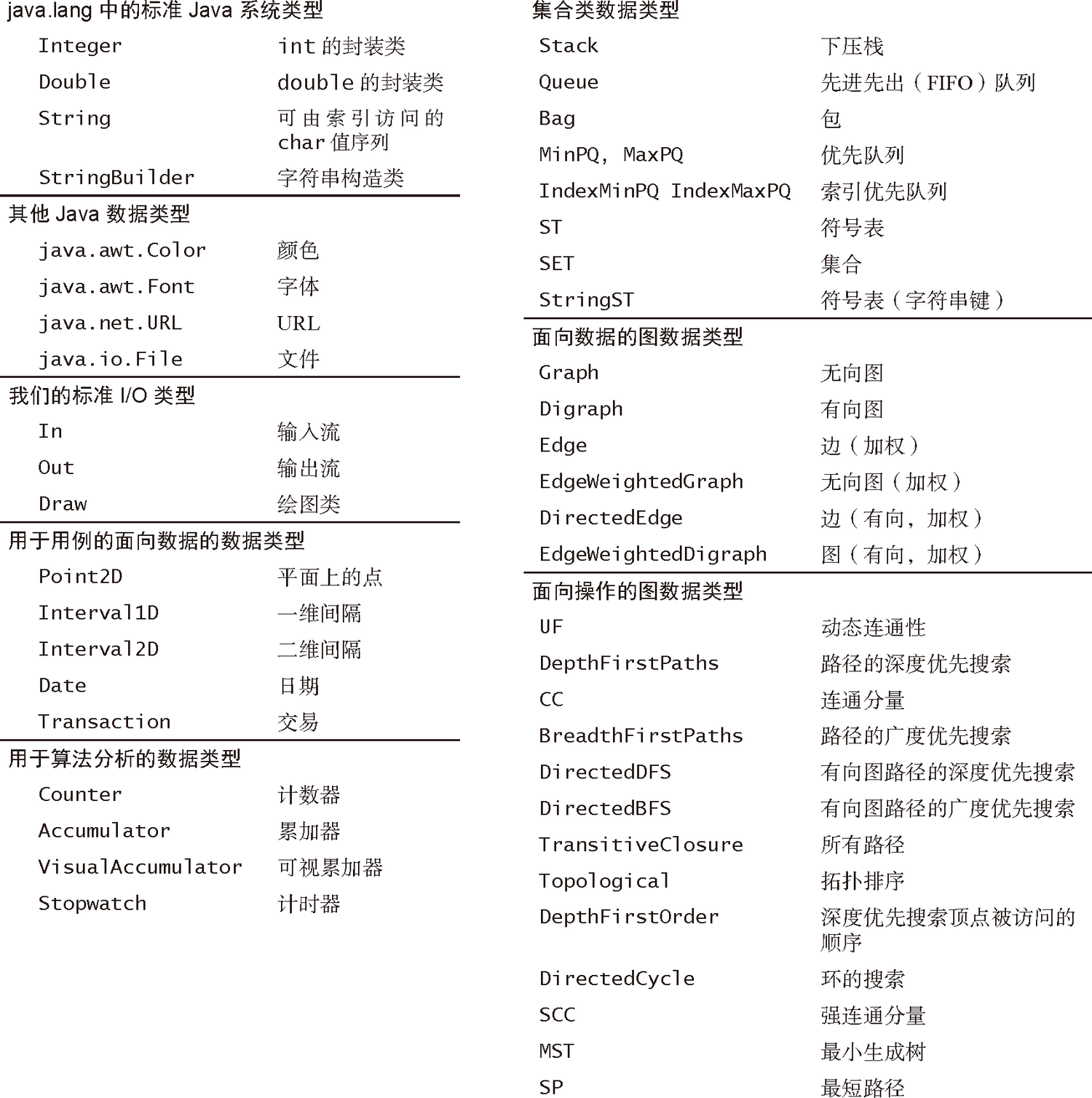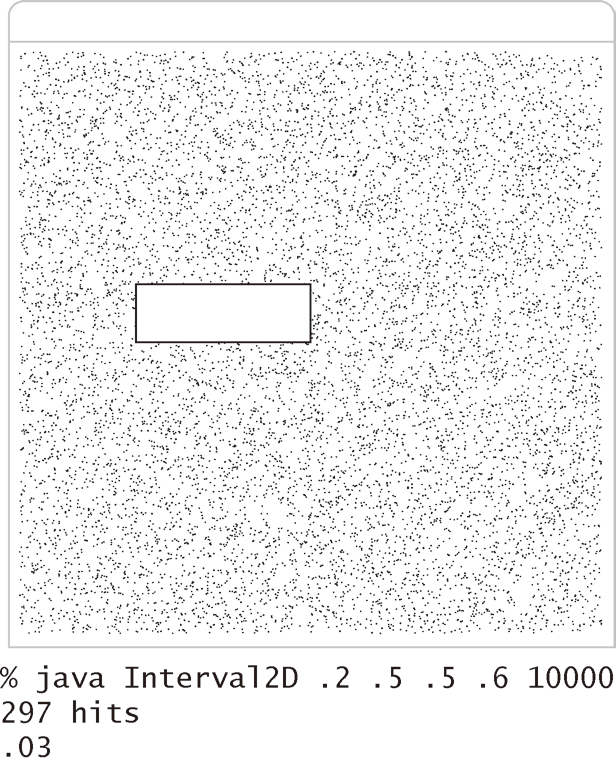# 算法（4th ed）(81)：基础——数据抽象 4.2.1(抽象数据类型举例：几何对象）public class Point2D               Point2D(double x, double y) 创建一个点      double   x() $x$ 坐标      double   y() $y$ 坐标      double   r() 极径（极坐标）      double   theta() 极角（极坐标）      double   distTo(Point2D that) 从该点到 that 的欧几里得距离        void   draw() 用 StdDraw 绘出该点

 public class Interval1D               Interval1D(double lo, double hi) 创建一个间隔       double  length() 间隔长度      boolean  contains(double x) x 是否在间隔中      boolean  intersect(Interval1D that) 该间隔是否和间隔 that 相交         void  draw() 用 StdDraw 绘出该间隔

 public class Interval2D           Interval2D(Interval1D x, Interval1D y) 创建一个二维间隔   double  area() 二维间隔的面积  boolean  contains(Point2D p) p 是否在二维间隔中  boolean  intersect(Interval2D that) 该间隔是否和二维间隔 that 相交     void  draw() 用 StdDraw 绘出该二维间隔

public static void main(String[] args){   double xlo = Double.parseDouble(args);   double xhi = Double.parseDouble(args);   double ylo = Double.parseDouble(args);   double yhi = Double.parseDouble(args);   int T = Integer.parseInt(args);    Interval1D xinterval = new Interval1D(xlo, xhi);   Interval1D yinterval = new Interval1D(ylo, yhi);   Interval2D box = new Interval2D(xinterval, yinterval);   box.draw();    Counter c = new Counter("hits");   for (int t = 0; t < T; t++)   {      double x = Math.random();      double y = Math.random();      Point2D p = new Point2D(x, y);      if (box.contains(p)) c.increment();      else                 p.draw();   }    StdOut.println(c);   StdOut.println(box.area());}
Interval2D 的测试用例